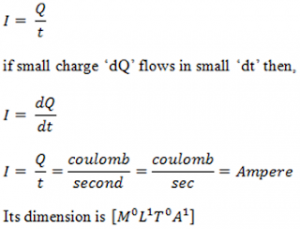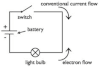# Current And Its Direction

Current
The rate of flow of charge in any specific direction is called current. If Q charge flows through a conductor in time t then current is:If one coulomb of charge flows through a conductor in one second then the current in the conductor is said to be one ampere.

Direction of Current:
Before the discovery of electron it was considered that current is due to the flow of positive charge. So, the direction of current was assumed to be from positive terminal of battery to the negative terminal of the battery. Later electron was discovered and it was obvious that current is due to the flow of electrons from negative terminal to the positive terminal of the battery. However, till date we assume the direction of current from positive terminal to the negative terminal. This direction of current is called conventional direction of current.Current carriers
In metals large number of free electrons are available. These free electrons carry negative charges. The flow of these charges contributes current. In case of electrolytes the positive and negative ions are responsible for current through them. In case of semiconductors the combined motion of electrons and hole gives current.

Types of current
1. DC (Direct current): The current whose magnitude and direction remains same throughout the flow of current is called DC.
eg: current due to a battery is DC current.

2. AC (Alternating current): The current whose magnitude and direction changes continuously and periodically is called AC current.
eg: current due to a AC generator is AC current